# NCERT Solutions for Class 12 Chemistry Chapter 9 Coordination Compounds

In this chapter, we provide NCERT Solutions for Class 12 Chemistry Chapter 9 Coordination Compounds for English medium students, Which will very helpful for every student in their exams. Students can download the latest NCERT Solutions for Class 12 Chemistry Chapter 9 Coordination Compounds pdf, free NCERT solutions for Class 12 Chemistry Chapter 9 Coordination Compounds book pdf download. Now you will get step by step solution to each question. Class 12 Chemistry is a very important subject for entrance exams like IIT JEE, CPMT, etc.

## NCERT Solutions for Class 12 Chemistry Chapter 9 Coordination Compounds

INTEXT Questions

Question 1.
Write the formulas for the following coordination compounds :

1. Tetraamminediaquacobalt (lll) chloride
2. Potassium tetracyanonickelate (ll)
3. Tris(ethane-1,2-diamine) chromium (lll) chloride
4. Amminebromidochloridonitrito-N- platinate (ll)
5. Dichloridobis(ethane-1,2-diamine) platinum (IV) nitrate
6. Iron (lll) hexacyanoferrate (ll)

Solution:Question 2.
Write the IUPAC names of the following coordination compounds :Solution:

1. Hexaamminecobalt (III) chloride
2. Pentaamminechloridocobalt (III) chloride (HO3)2
3. Potassium hexacyanoferrate (III)
4. Potassium trioxalatoferrate (III)
6. Diamminechlorido (methylamine) platinum (II) chloride

Question 3.
Indicate the types of isomerism exhibited by the following complexes and draw the structures for these isomers :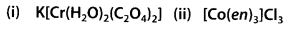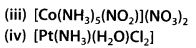Solution: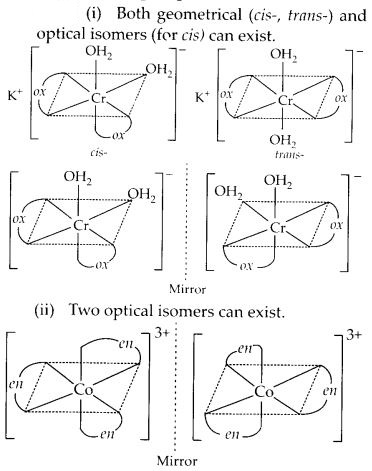Question 4.
Give evidence that [CO(NH3)5CI] SO4 and [CO(NH3)SO4]Cl are ionisation isomers.
Solution:
The ionisation isomers dissolve in water to yield different ions and thus react differently to various reagents :Question 5.
Explain on the basis of valence bond theory that [Ni(CN)4]2- ion with square planar structure is diamagnetic and the [NiCI4]2- ion with tetrahedral geometry is paramagnetic.

Solution: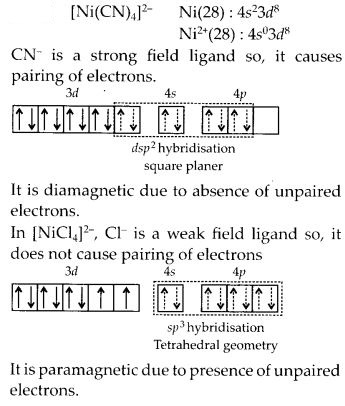Question 6.
[NiCl4]2- is paramagnetic while [Ni(CO)4] is diamagnetic though both are tetrahedral. Why?
Solution:
In Ni(CO)4, Ni is in zero oxidation state whereas in NiCl42-, it is in +2 oxidation state. In the presence of CO ligand, the unpaired d-electrons of Ni pair up but Cl being a weak ligand is unable to pair up the unpaired electrons.

Question 7.
[Fe(H2O)6]3+ is strongly paramagnetic whereas [Fe(CN)6]3- is weakly paramagnetic. Explain.
Solution:
In presence of CN, (a strong ligand), the 3d electrons pair up leaving only one unpaired electron. The hybridisation is dsp3 forming inner orbital complex. In the presence of H2O, (a weak ligand), 3d electrons do not pair up. The hybridisation is sp3 d2 forming an outer orbital complex containing five unpaired electrons hence, it is strongly paramagnetic.

Question 8.
Explain [CO(NH3)6]3+ is an inner orbital complex whereas [Ni(NH3)6]2+ is an outer orbital complex.
Solution:
in the presence of NH3, the 3d electrons pair up leaving two d orbitals empty to be involved in d2 sp3 hybridisation forming inner orbital complex in case of [CO(NH3)6]3+.
In Ni(NH3)6]2+, Ni is in +2 oxidation state and has d3 configuration, the hybridisation involved is sp3 d2 forming outer orbital complex.

Question 9.
Predict the number of unpaired electrons in the square planer [Pt(CN)4]2- ion.
Solution:
For square planer shape, the hybridisation is dsp2 . Hence, the unpaired electrons in 5d orbital pair up to make one f-orbital empty for dsp2 hybridisation. Thus there is no unpaired electron.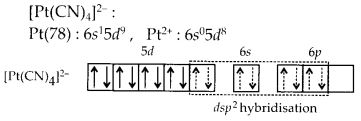Question 10.
The hexaaquomanganese (ll) ion contains five unpaired electrons, while the hexacyano ion contains only one unpaired electron. Explain using Crystal Field Theory.
Solution:
Mn(II) has 3d5 electronic configuration. Water is a weak field ligand and therefore ∆0 is small. Therefore, the hexaaqua complex will be high spin complex containing 5 unpaired electrons. On the other hand, CN is a strong field ligand and therefore, ∆0 is large. Therefore, in its cyano complex, the electrons pair up and have only one unpaired electron.Question 11.
Calculate the overall complex dissociation equilibrium constant for the Cu(NH3)42+ ion, given that β4 for this complex is 2.1 × 1013.

Solution: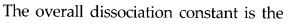NCERT Exercises

Question 1.
Explain the bonding in coordination compounds in terms of Werner’s postulates.
Solution:
The main postulates are :

1. In coordination compounds metals show two types of linkages (valencies)-primary and secondary.
2. The primary valencies are normally ionisable and are satisfied by negative ions.
3. The secondary valencies are non ionisable. These are satisfied by neutral molecules or negative ions. The secondary valency is equal to the coordination number and is fixed for a metal.
4. The ions/groups bound by the secondary linkages to the metal have characteristic spatial .arrangements corresponding to different coordination numbers.

Question 2.
FeSO4 solution mixed with (NH4)2SO4 solution in 1:1 molar ratio gives the test of Fe2+ ion but CuSO4 solution mixed with aqueous ammonia in 1:4 molar ratio does not give the test of Cu2+ ion. Explain why?
Solution:
FeSO4 solution when mixed with (NH4)2SO4 solution in 1:1 molar ratio forms a double salt FeSO4(NH4)2SO4-6H2O which when dissolved in water dissociates into simple ions to give tests for its constituent ions. When CuSO4 is mixed with aqueous ammonia a complex ion [Cu(NH3)4]2+ is formed which does not give Cu2+ in the solution.

Question 3.
Explain with two examples each of the following: coordination entity, ligand, coordination number, coordination polyhedron, homoleptic and heteroleptic.
Solution:
(i) Coordination entity : A coordination entity constitutes a central metal atom or ion bonded loa fixed number of ions or molecules. For example, [COCl3(NH3)3] is a coordination entity in which the cobalt ion is surrounded by three ammonia molecules and three chloride ions. Other examples are [Ni(CO)4], [PtCl2(NH3)2], [Fe(CN)6]4-, [CO(NH3)6]3+.

(ii) Ligand : The ions or molecules bound to the central atom/ion in the coordination entity are called ligands. These may be simple ions such as Cl, small molecules such as H2O or NH3, larger molecules such as H2NCH2CH2NH2 or N(CH2CH2NH2)3 or even macromolecules, such as proteins.

(iii) Coordination number : The coordination number [C.N.] of a metal ion in a complex can be defined as the number of ligand or donor atoms to which the metal is directly bonded. For example, in the complex ions, [PtCl6]2- and [Ni(NH3)4]2+, the coordination number of Pt and Ni are 6 and 4 respectively. Similarly, in the complex ions, [Fe(C2O4)3]3- and [CO(en)3]3+,the coordination number of both, Fe and Co, is 6 because C2O42-and en (ethane-1,2-diamine) are bidentate ligands.

(iv) Coordination polyhedron : The spatial arrangement of the ligand atoms which are directly attached to the central atom/ion defines a coordination polyhedron about the central atom. The most common coordination polyhedra are octahedral, square planar and tetrahedral. For example, [CO(NH3)6]3+ is octahedral, [Ni(CO)4] is tetrahedral and [PtCl4]2- is square planar.

(v) Homoleptic and heteroleptic complexe : Complexes in which a metal is bound to only one kind of donor groups, e.g., [CO(NH3)6]3+, are known as homoleptic. Complexes in which a metal is bound to more than one kind of donor groups, e.g., [CO(NH3)4Cl2]+, are known as heteroleptic.

Question 4.
What is meant by unidentate, bidentate and ambidentate ligands? Give two examples for each.
Solution:
Unidentate ligands are those which bind to the metal ion through a single donor atom, e.g., Cl , H2O.

Bidentate ligands are those which bind to the metal ion through two donor atoms. e.g., ethane-1,2-diamine (H2NCH2CH2NH2), oxalate (C2O42-) ion.

Ambidentate ligands are those which can bind to metal ion through two different donor atoms, e.g., NO2 and SCN ion.

Question 5.
Specify the oxidation numbers of the metals in the following coordination entities :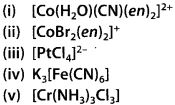Solution: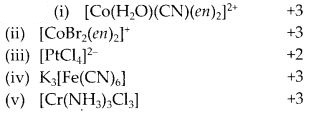Question 6.
Using IUPAC norms write the formulas for the following :

1. Tetrahydroxozincate (ll)
3. Diamminedichloridoplatinum (ll)
4. Potassium tetracyanonickelate (ll)
5. Pentaamminenitritio-O-cobalt (lll)
6. Hexaamminecobalt (lll) sulphate
7. Potassium tri(oxalato)chromate (lll)
8. Hexaammineplatinum (IV)
9. Tetrabromidocuprate (ll)
10. Pentaamminenitrito-N-cobalt (lll)

Solution: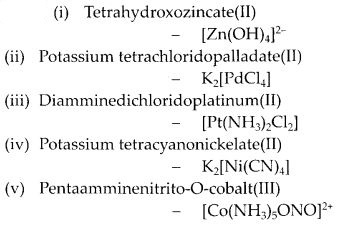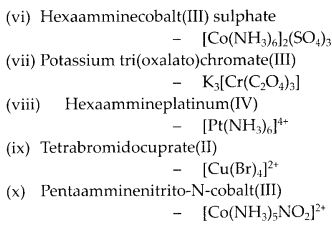Question 7.
Using IUPAC norms write the systematic names of the following :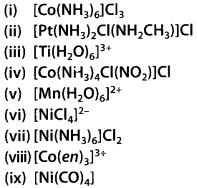Solution:

1. Hexaamminecobalt (III) chloride
2. Diamminechloridomethylamine platinum (II) chloride
3. Hexaaquatitanium (III) ion
4. Tetraamminechloridonitrito-N-cobalt (III) chloride
5. Hexaaquamanganese (II) ion
6. Tetrachloridonickelate (II) ion
7. Hexaammine nickel (II) chloride
8. Tris (ethane-1,2-diamine) cobalt(III) ion
9. Tetracarbonylnickel (0)

Question 8.
List various types of isomerism possible for coordination compounds, giving an example of each.
Solution:
(i) Geometrical isomerism : This type of isomerism arises in heteroleptic complexes due to different possible geometric arrangements of the ligands. Important examples of this behaviour are found with coordination numbers 4 and 6. In a square planar complex of formula [MX2L2] (X and L are unidentate), the two identical ligands may be arranged adjacent to each other in a cis- isomer, or opposite to each other in a trans isomer.

Another type of geometrical isomerism occurs in octahedral coordination entities of the type [Ma3b3] like [CO(NH3)3(NO2)3]. If three donor atoms of the same ligands occupy adjacent positions at the corners of an octahedral face, we have the facial (fac) isomer. When the positions are around the meridian of the octahedron, we get the meridional (mer) isomer.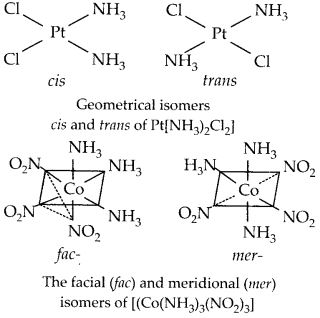(ii) Optical isomerism : Optical isomerism is common in octahedral complexes involving bidentate ligands. In a coordination entity of the [PtCl2(en)2]2+, only the ds-isomer shows optical activity.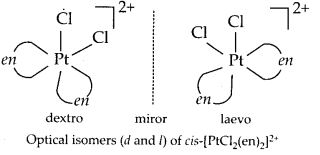(iii) Linkage isomerism : Linkage isomerism arises in a coordination compound containing ambidentate ligand. A simple example is provided by complexes containing the thiocyanate ligand, NCS, which may bind through the nitrogen to give M-N CS or through sulphur to give M-SCN. This behaviour was seen in the complex [CO(NH3)5(NO2)]Cl2, which is obtained as the red form, in which the nitrite ligand is bound through oxygen ( ONO), and as the yellow form, in which the nitrite ligand is bound through nitrogen (-NO2).

(iv) Coordination isomerism : This type of isomerism arises from the interchange of ligands between cationic and anionic entities of different metal ions present in a complex. An example is provided by [Co(NH3)6] [Cr(CN)6], in which the NH3 ligands are bound to CO3+ and the CN ligands to Cr3+. In its coordination isomer [Cr(NH3)6][CO(CN)6], the NH3 ligands are bound to Cr3+ and the CN ligands to CO3+.

(v) Ionisation isomerism : This form of isomerism arises when the counter ion in a complex salt is itself a potential ligand and can displace a ligand which can then become the counter ion. An example is provided by the ionisation isomers [CO(NH3)5SO4]Br and [CO(NH3)5Br]SO4.

(vi) Solvate isomerism : This form of isomerism is known as ‘hydrate isomerism in case where water is involved as a solvent. This is similar to ionisation isomerism. Solvate isomers differ by whether or not a solvent molecule is directly bonded to the metal ion or merely present as free solvent molecules in the crystal lattice. An example is provided by the aqua complex [Cr(H2O)6]Cl3 (violet) and its solvate isomer [Cr(H2O)5Cl]Cl2 H2O (grey green).

Question 9.
How many geometrical isomers are possible in the following coordination entities?

1. [Cr(C2O4)3]3-
2. [CO(NH3)3CI3]

Solution:

1. Zero
2. Two – Facial and meridional.

Question 10.
Solution:

Solution: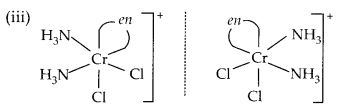Question 11.
Draw all the isomers (geometrical and optical) of :Solution: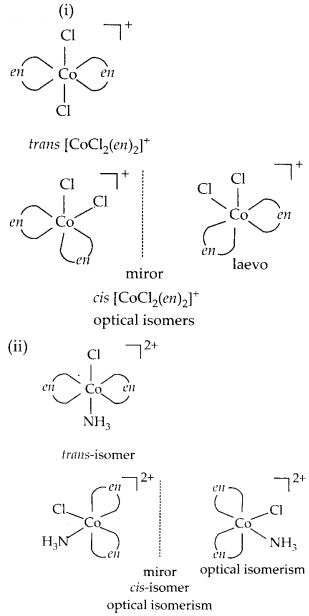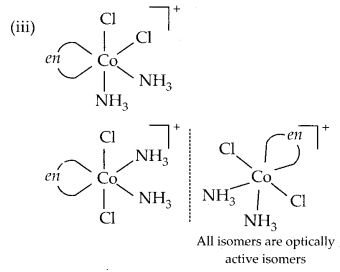Question 12.
Write all the geometrical isomers of [Pt(NH3)(Br)(CI)(py)] and how many of these will exhibit optical isomerism?

Solution: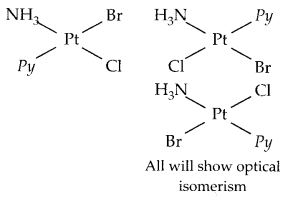Question 13.
Aqueous copper sulphate solution (blue in colour) gives :

1. a green precipitate with aqueous potassium fluoride and
2. a bright green solution with aqueous potassium chloride. Explain these experimental results.

Solution: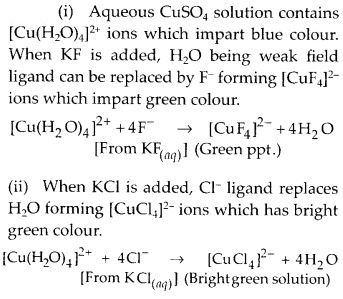Question 14.
What is the coordination entity formed when excess of aqueous KCN is added to an aqueous solution of copper sulphate? Why is it that no precipitate of copper sulphide is obtained when H2S(g) is passed through this solution?
Solution:

CN is a strong field ligand, therefore, [Cu(CN)4]2- is highly stable and has large value of stability constant. On passing H2S, CuS is not formed because this coordination entity does not give Cu2+ ion.

Question 15.
Discuss the nature of bonding in the following coordination entities on the basis of valence bond theory :Solution: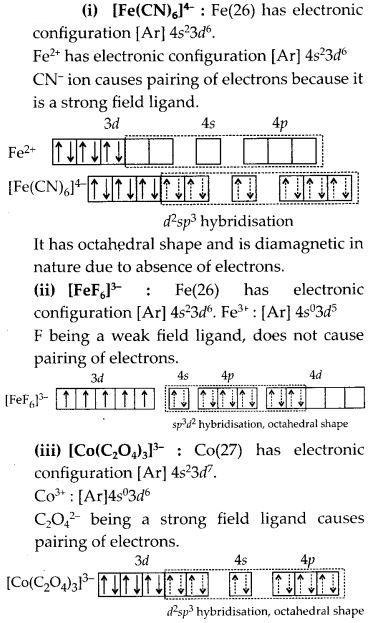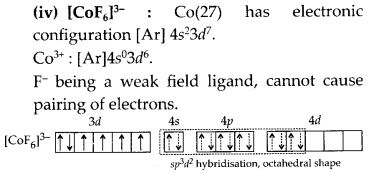Question 16.
Draw figure to show the splitting of d-orbitals in an octahedral crystal field.
Solution:
Let us assume that the six ligands are positioned symmetrically along the Cartesian axes, with metal atom at the origin.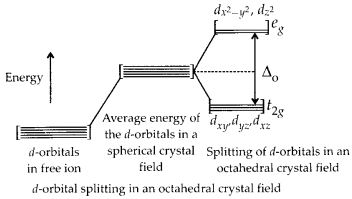As the ligands approach, first there is an increase in energy of d-orbitals relative to that of the free ion just as would be the case in a spherical field.

The orbitals lying along the axes (dz2 and dx2 – y2) get repelled more strongly than dxy, dyz and dzx orbitals which have lobes directed between the axes.

The dz2 and dx2-y2 orbitals get raised in energy and dxy dyz, dxz orbitals are lowered in energy relative to the average energy in the spherical crystal field. Thus, the degenerate set of d-orbitals get split into two sets : the lower energy orbitals set, t2g and the higher energy orbitals set, eg. The energy is separated by ∆0

Question 17.
What is spectrochemical series? Explain the difference between a weak field ligand and a strong field ligand.
Solution:
The crystal field splitting, ∆0, depends upon the field produced by the ligand and charge on the metal ion. Some ligands are able to produce strong fields in which, the splitting will be large whereas others produce weak fields and consequently result in small splitting of d-orbitals. In general, ligands can be arranged in a series in the order of increasing field strength as given below :Such a series is termed as spectrochemical series.

If ∆0 < P, the fourth electron enters one of the eg orbitals giving the configuration t23g g e1g. Ligands for which ∆0 < P are known as weak field ligands and form high spin complexes. If ∆0 > P, it becomes more energetically favourable for the fourth electron to occupy a t2g orbital with configuration t2g 4e0g. Ligands which produce this effect are known as strong field ligands and form low spin complexes.

Question 18.
What is crystal field splitting energy? How does the magnitude of ∆0 decide the actual configuration of d-orbitals in a coordination entity?

Solution: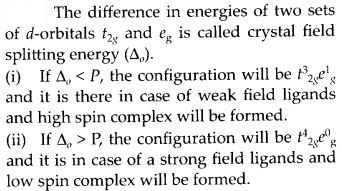Question 19.
[Cr(NH3)6]3+ is paramagnetic while [Ni(CN)4]2- is diamagnetic. Explain why?

Solution:Question 20.
A solution of [Ni(H2O)6]2+ is green but a solution of [Ni(CN)4]2- is colourless. Explain.
Solution:
[Ni(H2O)6]2+has unpaired electrons due to weak H2O ligands which absorb light from visible region and radiate complementary colour i.e., green whereas [Ni(CN)4]2- does not have any unpaired electron due to strong CN ligand, therefore, does not absorb light from visible region hence, it is colourless.

Question 21.
[Fe(CN)6]4- and [Fe(H2O)6]2+ are of different colours in dilute solutions. Why?

Solution: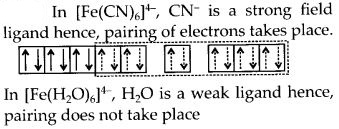Both ligands show different magnitude of crystal field splitting energy due to different nature hence, absorb different wavelengths ans show different colours.

Question 22.
Discuss the nature of bonding in metal carbonyls.
Solution:

The metal-carbon bond in metal carbonyls possess both s and p character. The M-C σ bond is formed by the donation of lone pair of electrons on the carbonyl carbon into a vacant orbital of the metal. The M-C π bond is formed by the donation of a pair of electrons from a filled d-orbital of metal into the vacant antibonding π* orbital of carbon monoxide. The metal to ligand bonding creates a synergic effect which strengthens the bond between CO and the metal.

Question 23.
Give the oxidation state, d-orbital occupation and coordination number of the central metal ion in the following complexes :Solution:Question 24.
Write down the IUPAC name for each of the following complexes and indicate the oxidation state,electronic configuration and coordination number. Also give stereochemistry and magnetic moment of the complex :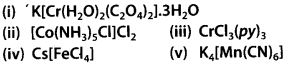Solution: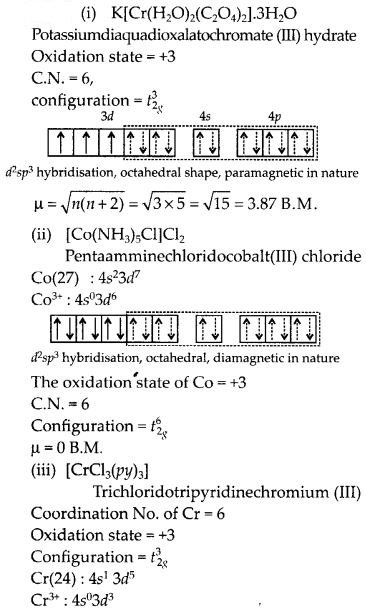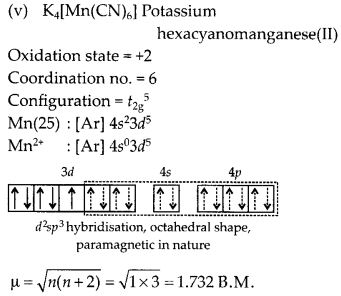Question 25.
What is meant by stability of the coordination compound in solution? State the factors which govern stability of complexes.
Solution:
The stability of a complex in solution refers to the degree of association between the two species involved in the state of equilibrium. The magnitude of the (stability or formation) equilibrium constant for the association, quantitatively expresses the stability. Thus, if we have a reaction of the type :then, larger the stability constant, the higher is the proportion of ML4 that exists in solution. Free metal ions rarely exist in the solution so that M will usually be surrounded by solvent molecules which will compete with the ligand molecules, L and be successively replaced by them. For simplicity, we generally ignore these solvent molecules and write four stability constants as follows :Factors affecting stability of complexes :

1. Smaller the size of cation, greater will be the stability of complex e.g., Fe3+ forms more stable complex than Fe2+.
2. Greater the charge on central metal ion, more stable will be the complex e.g., Pt4+ forms more stable complex than Pt2+.
3. Stronger the ligand, more stable will be the complex formed e.g., CN forms more stable complex then NH3.

Question 26.
What is meant by the chelate effect1 Give an example.
Solution:
When a di-or polydentate ligand uses its two or more donor atoms to bind a single metal ion, it is said to be a chelate ligand. The number of such ligating groups is called the denticity of the ligand. Such complexes, called chelate complexes tend to be more stable than similar complexes containing unidentate ligands. Example : EDTA, DMG, etc.

Question 27.
Discuss briefly giving an example in each case the role of coordination compound in :

1. biological systems
2. medicinal chemistry
3. analytical chemistry
4. extraction/metallurgy of metals.

Solution:
(i) Coordination compounds are of great importance in biological systems. The pigment responsible for photosynthesis, chlorophyll, is a coordination compound of magnesium. Haemoglobin, the red pigment 1 of blood which acts as oxygen carrier is a coordination compound of iron. Vitamin B12, cyanocobalamine, the anti- pernicious anaemia factor, is a coordination compound of cobalt. Among the other compounds of biological importance with coordinated metal ions are the enzymes like, carboxypeptidase A and carbonic anhydrase (catalysts of biological systems).

(ii) There is growing Interest in the use of chelate therapy in medicinal chemistry. An example is the treatment of problems caused by the presence of metals in toxic proportions in plant/animal systems. Thus, excess of copper and iron are removed by the chelating ligands D-penicillamine and desferrioxime B via the formation of coordination compounds.

EDTA is used in the treatment of lead poisoning. Some coordination compounds of platinum effectively inhibit the growth of tumours. Examples are: ds-platin and related compounds.

(iii) Coordination compounds find use in many qualitative and quantitative chemical analysis. The familiar colour reactions given by metal ions with a number of ligands (especially chelating ligands), as a result of formation of coordination entities, form the basis for their detection and estimation by classical and instrumental methods of analysis. Examples of such reagents include EDTA, DMG (dimethylglyoxime), a-nitroso- β-naphthol, cupron, etc.

(iv) Some important extraction processes of metals, like those of silver and gold, make use of complex formation. Gold, for example, combines with cyanide in the presence of oxygen and water to form the coordination entity [Au(CN)2]- in aqueous solution. Gold can be separated in metallic form from this solution by the addition of zinc.

Question 28.
How many ions are produced from the complex CO(NH3)6CI2 in solution?

(a) 6
(b) 4
(c) 3
(d) 2

Solution:Question 29.
Amongst the following ions which one has the highest magnetic moment value?

(a) [Cr(H2O)6]3+
(b) [Fe(H2O)6]2+
(c) [Zn(H2O)6]2+

Solution:Question 30.
The oxidation number of cobalt in K[Co(CO)4] is
(a) +1
(b) +3
(c) -1
(d) -3
Solution:
(c) : + 1 + x + 4(0) = 0
∴ x = – 1.

Question 31.
Amongst the following, the most stable complex is
(a) [Fe(H2O)6]3+
(b) [Fe(NH3)6]3+
(c) [Fe(C2O4)3]3-
(d) [FeCI6]3-
Solution:
(c) : Since C2O42- is a bidentate ligand, it forms the most stable complex.

Question 32.
What will be the correct order for the wavelengths of absorption in the visible region for the following :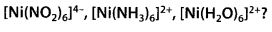Solution:
In spectrochemical series the order of the given ligands is H2O < NH3 < NO2– . Hence, the wave length of light will be absorbed in the opposite order since E =$\frac { hc }{ \lambda }$All Chapter NCERT Solutions For Class12 Chemistry

—————————————————————————–

All Subject NCERT Solutions For Class12

*************************************************

Remark:

I think you got complete solutions for this chapter. If You have any queries regarding this chapter, please comment on the below section our subject teacher will answer you. We tried our best to give complete solutions so you got good marks in your exam.

If these solutions have helped you, you can also share Careerkundali.in to your friends.

Best of Luck!!